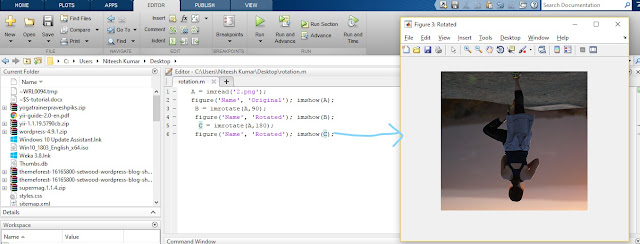All rights are reserved by Niteesh Kumar.. Theme images by Storman. Powered by Blogger.

# Image rotation in MATLAB

MATLAB is a high-performance language for technical computing. It integrates computation, visualization, and programming in an easy-to-use environment where problems and solutions are expressed in familiar mathematical notation. Typical uses include- Application development, including Graphical User Interface building.

MATLAB also provides image processing toolbox which is used to perform multiple operations in an image such as image processing, image analysis, image visualization and various algorithmic development. We can perform image segmentation, image enhancement, noise reduction, 3D image processing, image registration and geometric image transformation.

We can also automate common workflows such as rotation of image, reducing size of image, reducing pixel size of image and various other tasks related to image processing.

Here we are going to talk about how to rotate an image to any particular angle with the help of MATLAB image processing toolbox. We will first read the image and after that we will display the original image and then we will rotate image to 90 degree and then 180 degree.

Before doing that we need to know about few MATLAB commands-

Used to read the image from the specified path here format is optional, if we will specify the format then image will be read in specified format otherwise it will be read as it's original format which can be RGB or grayscale.

### imshow('image')

This displays the image in a figure window.

if we use multiple imshow() command to display multiple images then only last image will be shown as all the images will appear in the same figure window, to make all the images display in different windows we will need to learn figure() command.

### figure('property of window', 'name of the window')

There are multiple properties for a figure window here we are using Name property with which we are showing the original image as well as rotated image.

### imrotate('image', angle of rotation)

This MATLAB command is used to rotate an image with the specified value of rotation angle.

MATLAB program to rotate an image-

%reading image 2.png in variable A

%showing original image in a window named original

figure('Name', 'Original'); imshow(A);
%rotationg image with 90 degree
B = imrotate(A,90);
%showing 90 degree rotated image in a window named rotated
figure('Name', 'Rotated'); imshow(B);
%rotationg image with 90 degree
C = imrotate(A,180);
%showing 180 degree rotated image in a window named rotated
figure('Name', 'Rotated'); imshow(C);Original imageImage with 90 degree rotationImage with 18 degree rotation